# NEET Previous Year Paper 2010 with Answer Key

## NEET Previous Year Question Paper 2010 Main

91. Among the elements Ca, Mg, P and Cl, the order of increasing atomic radii is

(1) Mg < Ca < Cl < P                 (2) Cl < P < Mg < Ca

(3) P < Cl < Ca < Mg                 (4) Ca < Mg < P < Cl

Chemistry Notes

Physics Notes

Biology Notes

92. The reaction

2A(g) + B(g) ⇌ 3C(g) + D(g)

is begun with the concentrations of A and B both at an initial value of 1.00 M. When equilibrium is reached, the concentration of D is measured and found to be 0.25 M. The value for the equilibrium constant for this reaction is given by the expression

(1) [(0.75)3(0.25)] ÷ [(1.00)2(1.00)]

(2) [(0.75)3(0.25)] ÷ [(0.50)2(0.75)]

(3) [(0.75)3(0.25)] ÷ [(0.50)2(0.25)]

(4) [(0.75)3(0.25)] ÷ [(0.75)2(0.25)]

93.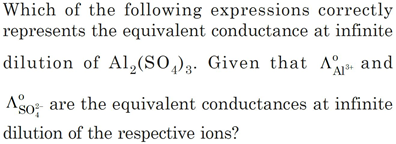(a)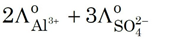(b)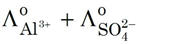(c)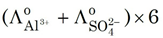(d)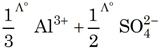94. The pressure exerted by 6.0 g of methane gas in a 0.03 m3 vessel at 129°C is (Atomic masses : C = 12.01, H = 1.01 and R = 8.314 JK–1 mol–1)

(1) 215216 Pa                  (2) 13409 Pa

(3) 41648 Pa                    (4) 31684 Pa

95.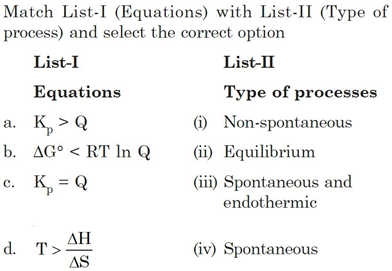(1) a – (i), b – (ii), c – (iii), d – (iv)

(2) a – (iii), b – (iv), c – (ii), d – (i)

(3) a – (iv), b – (i), c – (ii), d – (iii)

(4) a – (ii), b – (i), c – (iv), d – (iii)

Chemistry Notes

Physics Notes

Biology Notes

96. Among the following four compounds

a. Phenol                          b. Methyl phenol

c. Metanitrophenol           d. Paranitrophenol

The acidity order is

(1) d > c > a > b               (2) c > d > a > b

(3) a > d > c > b               (4) b > a > c > d

97. Among the following which one has the highest cation to anion size ratio?

(1) CsI                             (2) CsF

(3) LiF                             (4) NaF

98. Three moles of an ideal gas expanded spontaneously into vacuum. The work done will be

(1) Infinite                       (2) 3 Joules

(3) 9 Joules                      (4) Zero

Chemistry Notes

Physics Notes

Biology Notes

98. Three moles of an ideal gas expanded spontaneously
into vacuum. The work done will be

(1) Infinite                       (2)
3 Joules

(3) 9 Joules                      (4)
Zero

99. Which of the following species is not electrophilic in nature?

(1) Cl                  (2) BH3

(3) H3O               (4) NO2

100. A 0.66 kg ball is moving with a speed of 100 m/s. The associated wavelength will be

(h = 6.6 × 10–34 Js)

(1) 6.6 × 10–32 m                       (2) 6.6 × 10–34 m

(3) 1.0 × 10–35 m                       (4) 1.0 × 10–32 m

101. Consider the following relations for emf of a electrochemical cell

(a) emf of cell = (Oxidation potential of anode) – (Reduction potential of cathode)

(b) emf of cell = (Oxidation potential of anode) + (Reduction potential of cathode)

(c) emf of cell = (Reductional potential of anode) + (Reduction potential of cathode)

(d) emf of cell = (Oxidation potential of anode) – (Oxidation potential of cathode)

Which of the above relations are correct? Options:

(1) (c) and (a)                  (2) (a) and (b)

(3) (c) and (d)                  (4) (b) and (d)

Chemistry Notes

Physics Notes

Biology Notes

102. In which of the following molecules the central atom does not have sp3 hybridization?

(1) CH4                            (2) SF4

(3) BF4                           (4) NH4+

103. For vaporization of water at 1 atmospheric pressure, the values of ΔH and ΔS are 40.63 kJ mol–1 and 108.8 JK–1 mol–1, respectively. The temperature when Gibbs energy change (ΔG) for this transformation will be zero, is

(1) 273.4 K                      (2) 393.4 K

(3) 373.4 K                      (4) 293.4 K

104. Match List-I (substances) with List-II (process) employed in the manufacture of the substances and select the correct option

List-I                                          List-II

Substances                                 Processes

a. Sulphuric acid                        (i) Haber’s Process

b. Steel                                       (ii) Bessemer’s Process

c. Sodium hydroxide                 (iii) Leblanc Process

d. Ammonia                               (iv) Contact Process

(1) a – (i), b – (iv), c – (ii), d – (iii)

(2) a – (i), b – (ii), c – (iii), d – (iv)

(3) a – (iv), b – (iii), c – (ii), d – (i)

(4) a – (iv), b – (ii), c – (iii), d – (i)

105. When glycerol is treated with excess of HI, it produces

(1) 2-iodopropane           (2) Allyl iodide

(3) Propene                      (4) Glycerol triiodide

106. Some statements about heavy water are given below

a. Heavy water is used as a moderator in nuclear reactors

b. Heavy water is more associated than ordinary water

c. Heavy water is more effective solvent than ordinary water

Which of the above statements are correct?

(1) a and b                       (2) a, b and c

(3) b and c                       (4) a and c

107. The compound A on heating gives a colourless gas and a residue that is dissolved in water to obtain B. Excess of CO2 is bubbled through aqueous solution of B, C is formed which is recovered in the solid form. Solid C on gentle heating gives back A. The compound is

(1) CaCO3                                  (2) Na2CO3

(3) K2CO3                                  (4) CaSO4.2H2O

108.